Albert Einstein: Relativity
Part I: The Special Theory of Relativity

### The Behaviour of Measuring-Rods and Clocks in Motion

Place a metre-rod in the x1-axis of K1 in such a manner that one end (the beginning) coincides with the point x1=0 whilst the other end (the end of the rod) coincides with the point x1=I. What is the length of the metre-rod relatively to the system K? In order to learn this, we need only ask where the beginning of the rod and the end of the rod lie with respect to K at a particular time t of the system K. By means of the first equation of the Lorentz transformation the values of these two points at the time t = 0 can be shown to be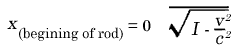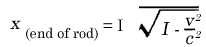the distance between the points being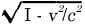.

But the metre-rod is moving with the velocity v relative to K. It therefore follows that the length of a rigid metre-rod moving in the direction of its length with a velocity v isof a metre.

The rigid rod is thus shorter when in motion than when at rest, and the more quickly it is moving, the shorter is the rod. For the velocity v=c we should have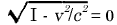,

and for stiII greater velocities the square-root becomes imaginary. From this we conclude that in the theory of relativity the velocity c plays the part of a limiting velocity, which can neither be reached nor exceeded by any real body.

Of course this feature of the velocity c as a limiting velocity also clearly follows from the equations of the Lorentz transformation, for these became meaningless if we choose values of v greater than c.

If, on the contrary, we had considered a metre-rod at rest in the x-axis with respect to K, then we should have found that the length of the rod as judged from K1 would have been;

this is quite in accordance with the principle of relativity which forms the basis of our considerations.

A Priori it is quite clear that we must be able to learn something about the physical behaviour of measuring-rods and clocks from the equations of transformation, for the magnitudes z, y, x, t, are nothing more nor less than the results of measurements obtainable by means of measuring-rods and clocks. If we had based our considerations on the Galileian transformation we should not have obtained a contraction of the rod as a consequence of its motion.

Let us now consider a seconds-clock which is permanently situated at the origin (x1=0) of K1. t1=0 and t1=I are two successive ticks of this clock. The first and fourth equations of the Lorentz transformation give for these two ticks :

t = 0

and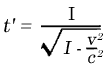As judged from K, the clock is moving with the velocity v; as judged from this reference-body, the time which elapses between two strokes of the clock is not one second, but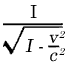seconds, i.e. a somewhat larger time. As a consequence of its motion the clock goes more slowly than when at rest. Here also the velocity c plays the part of an unattainable limiting velocity.